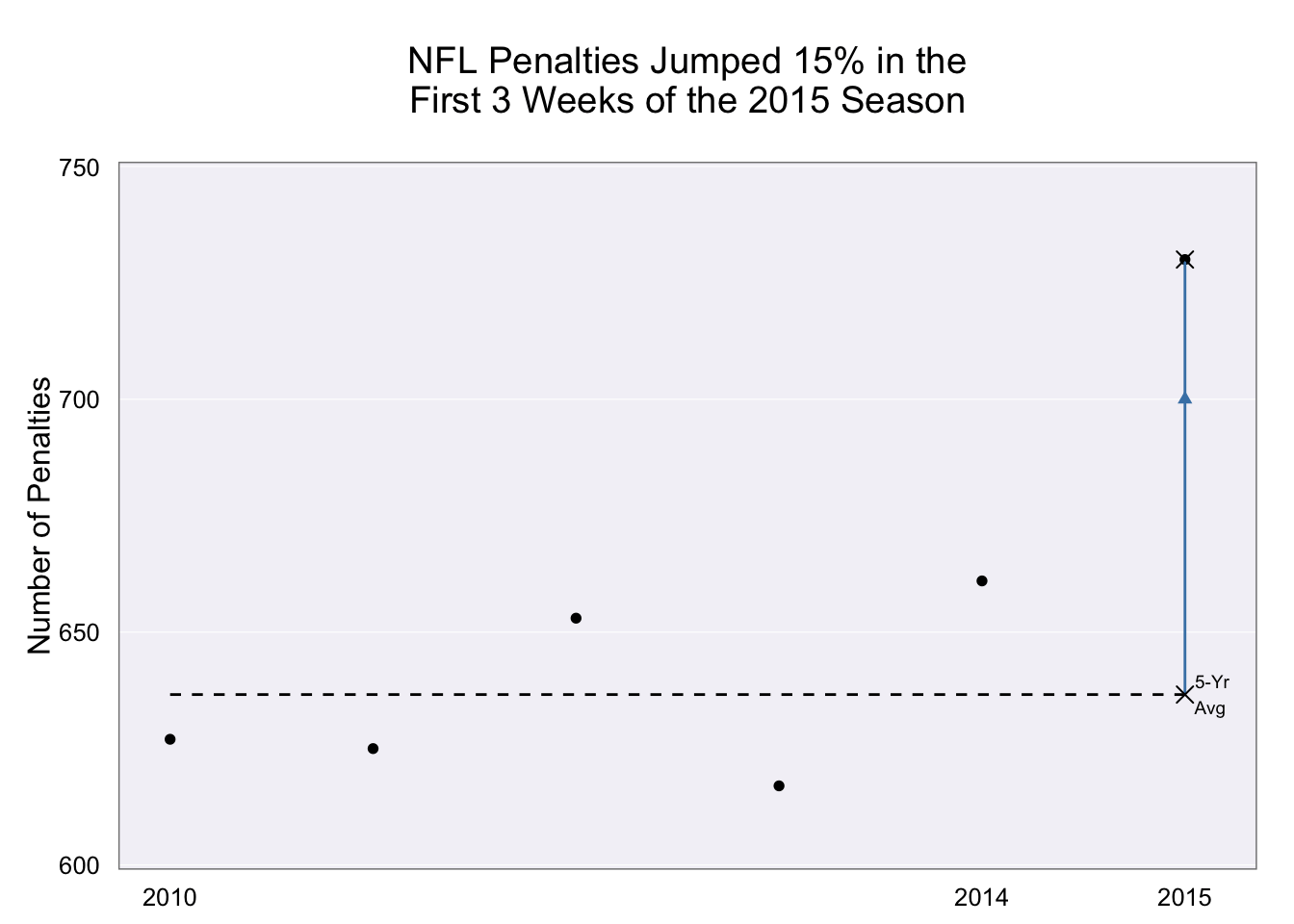# For Some Definition of “Directly” and/or “Contort”_”cannot be produced directly from a tool (without contorting your body in various painful locations)”_.

I beg to differ.

With R & ggplot2, I get to both pick my tool and design at the same time since I have a very flexible and multi-purpose tool. I also don’t believe that the code below qualifies as “contortions”, though I am a ggplot2 fanboi. It’s no different than Excel folks clicking on radio buttons and color pickers, except my process is easily repeatable & scalable once finalized (this is not finalized as it’s not 100% parameterized but it’s not difficult to do that last part).

```library(ggplot2)

dat <- data.frame(year=2010:2015,
penalties=c(627, 625, 653, 617, 661, 730))

last=dat\$penalties,
lab="5-Yr\nAvg")

gg <- ggplot(dat, aes(x=year, y=penalties))
gg <- gg + geom_point()
gg <- gg + scale_x_continuous(breaks=c(2010, 2014, 2015), limits=c(NA, 2015.1))
gg <- gg + scale_y_continuous(breaks=c(600, 650, 700, 750),
limits=c(599, 751), expand=c(0,0))
gg <- gg + geom_segment(data=avg, aes(x=2010, xend=2015, y=val, yend=val), linetype="dashed")
gg <- gg + geom_segment(data=avg, aes(x=2015, xend=2015, y=val, yend=last), color="steelblue")
gg <- gg + geom_point(data=avg, aes(x=2015, y=val), shape=4, size=3)
gg <- gg + geom_text(data=avg, aes(x=2015, y=val), label="5-Yr\nAvg", size=2.5, hjust=-0.3)
gg <- gg + geom_point(data=avg, aes(x=2015, y=700), shape=17, col="steelblue")
gg <- gg + geom_point(data=avg, aes(x=2015, y=730), shape=4, size=3)
gg <- gg + labs(x=NULL, y="Number of Penalties",
title="NFL Penalties Jumped 15% in the\nFirst 3 Weeks of the 2015 Season\n")
gg <- gg + theme_bw()
gg <- gg + theme(panel.grid.minor=element_blank())
gg <- gg + theme(panel.grid.major.x=element_blank())
gg <- gg + theme(panel.grid.major.y=element_line(color="white"))
gg <- gg + theme(panel.background=element_rect(fill="#f3f2f7"))
gg <- gg + theme(axis.ticks=element_blank())
gg```Amazon Author Page

## 5 Comments →For Some Definition of “Directly” and/or “Contort”

1.David Hugh-Jones

I think this ought to be a canonical case of when not to use ggplot2.

Why not just the much more readable

“`
dat <- data.frame(year=2010:2015,
penalties=c(627, 625, 653, 617, 661, 730))

m <- mean(dat\$penalties[1:5])
last <- dat\$penalties

plot(penalties ~ year, data = dat,
xlim = c(2010, 2015.5), ylim = c(600, 750),
pch = 19, tck = 0, xaxt = “n”,
ylab = “Number of penalties”, xlab = “Year”,
main = “NFL Penalties Jumped 15% in the\nFirst 3 Weeks of the 2015 Season”)
axis(1, c(2010, 2014, 2015), labels = TRUE, tick = FALSE)
segments(x0 = 2010, x1 = 2015, y0 = m, lty = 2)
text(2015.2, m + 2, “5-Yr\nAvg”)
segments(x0 = 2015, y0 = m, y1 = last)
arrows(x0 = 2015, y0 = m, y1 = last * 2/3 + m * 1/3, length = 0.15)
points(c(2015, 2015), c(m, last), pch = 4, cex = 1.5)
“`

1.hrbrmstr

missing colors; white y major ticks (once you add colors); proper orientation for y tick labels; arrow shape is not the same (at least do apple variety to apple variety). plus, no arcane pch, tck & xact incantations :-) with some optimization and a valid 1:1 comparison, I can get the ggplot # of lines to match or come close to matching yours, too.

but, you have proved juncharts wrong as well! which was the whole point of the post.

2.Fang Xianfu

I get that the idea was to reproduce the original… but the arrow head in the middle of the line is horrible :( Fixing that would also allow you to remove your geompoint for the arrowhead and just add the arrow param to your geomsegment, simplifying and beautifying it at the same time :)

1.hrbrmstr

Totally agreed regarding the aesthetics. I think he was going for a “football playbook” feel. If that’s the case I’d’ve changed up a whole lot more (prbly even use hollow points). If only WordPress sites took pull requests :-)

This site uses Akismet to reduce spam. Learn how your comment data is processed.